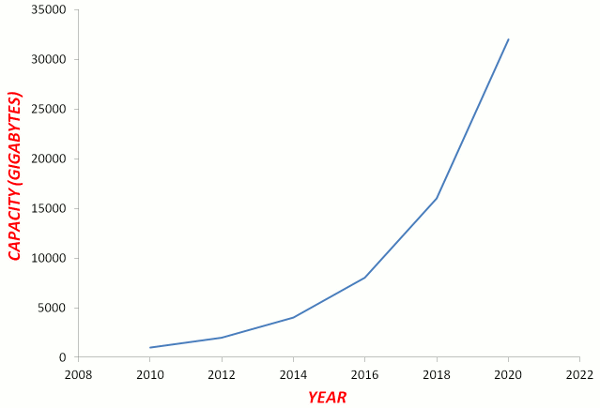# Line Graphs

Line Graphs show continuous data. Examples include the world population, the value of the dollar, average daily temperatures, a patient's temperatures, and distances travelled on a journey.

Of the two variables in bar graphs, the independent variable is the one on which the other variable depends. The independent variable goes on the horizontal axis and a common example is the months of the year.

The dependent variable is written on the vertical axis and a common example includes the frequency.

Some important tips are:

• The numbers that are used on the axes should go up by 1s, 2s, 5s, 10s, 20s, 50s, 100s, 200s, 500s, 1000s and so on.
• Never use other numbers such as 3s, 4s, 6s, 7s, 8s and 9s.
• The axes do not have to start at zero.

## Example - Computing Power

Moore's Law of Computing Power states that the number of components in integrated circuits (associated with processing speed, memory capacity, and the number of pixels in cameras) doubles every 2 years.

 YEAR(INDEPENDENT VARIABLE) CAPACITY(DEPENDENT VARIABLE) 2010 1 000 gigabytes 2012 2 000 gigabytes 2014 4 000 gigabytes 2016 8 000 gigabytes 2018 16 000 gigabytes 2020 32 000 gigabytes